# 带源项浅水波方程的高分辨率熵稳定格式*

(长安大学 理学院， 西安 710064)

## 引 言

Ut+F(U)x+G(U)y=S(x,y,U)，

(1)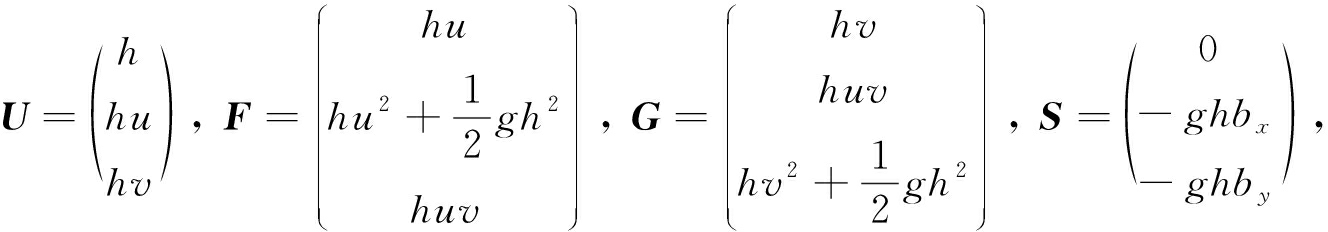h为水深，u,v分别为xy方向的水流速度，g为重力加速度，函数b=b(x,y)为底部地势在没有源项的情况下，浅水波方程是一类非线性的双曲守恒律方程非线性双曲守恒律方程有一个显著的特点是：不管初始条件是否光滑，其数值解都可能随时间的推移在某一时刻产生间断这对求解浅水波方程的精确解带来了很大的困难1954年，Lax针对双曲守恒律方程提出了弱解的概念，在弱意义下允许间断解的存在；但是弱解并不唯一为了确定具有物理意义的唯一弱解，1973年Lax又提出了熵稳定条件文献把熵稳定条件推广到了带源项浅水波方程，即如果弱解U在弱意义下满足熵不等式：

E(U)t+(H(U)+J1)x+(K(U)+J2)y≤0，

UH=〈V,∂UF(U)〉， ∂UK=〈V,∂UG(U)〉， ∂xJ1+∂yJ2=〈V,S〉，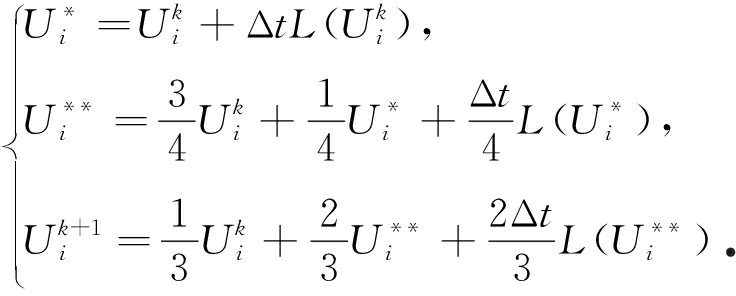## 1 一维浅水波方程求解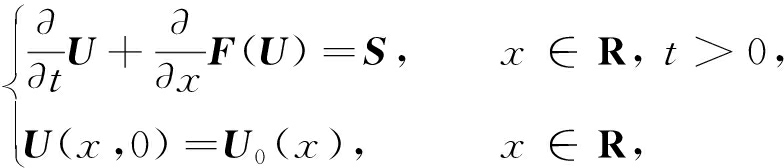(2)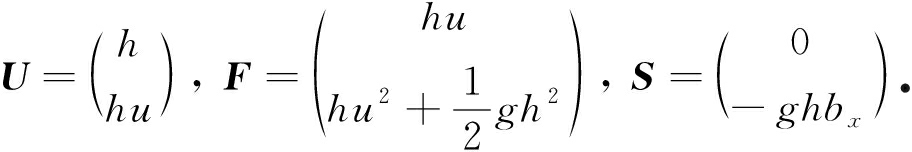### 1.1熵守恒格式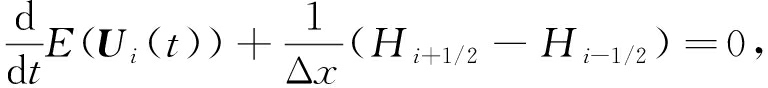(3)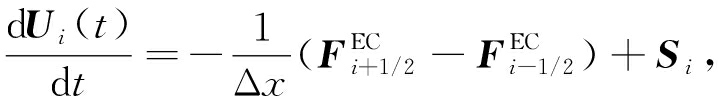(4)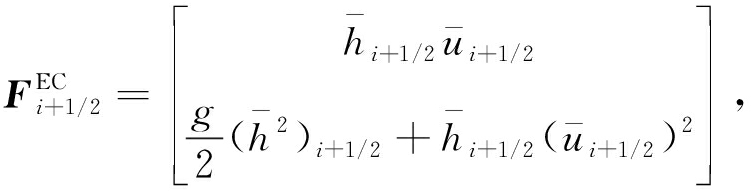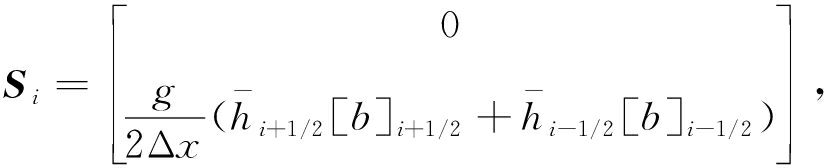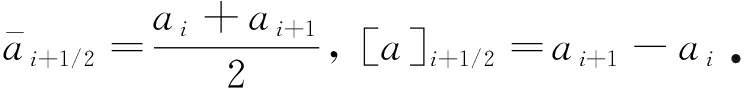### 1.2熵稳定格式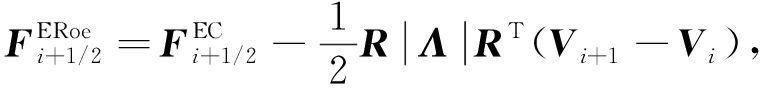(5)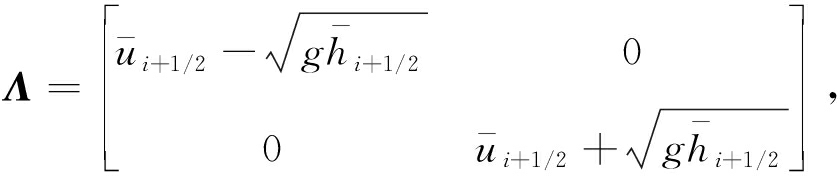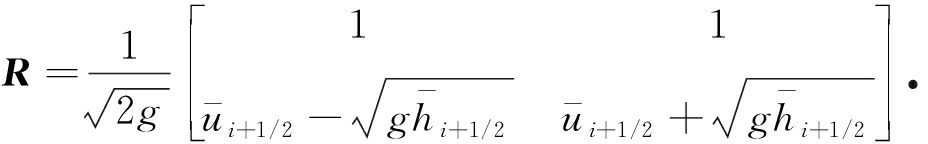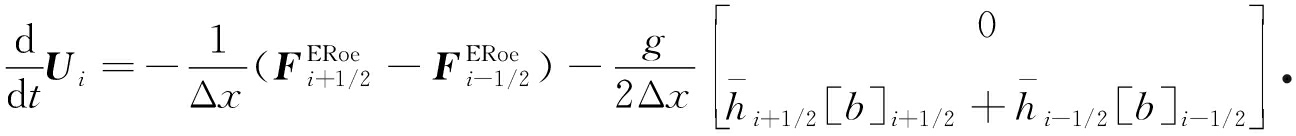(6)

### 1.3基于限制器的熵稳定格式

φ(θ)=max(0,min(1,2θ)，min(1,θ))

ψ1=diag(φ(θ1),φ(θ2))，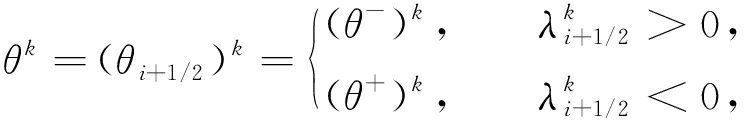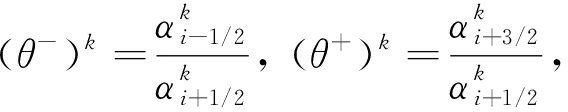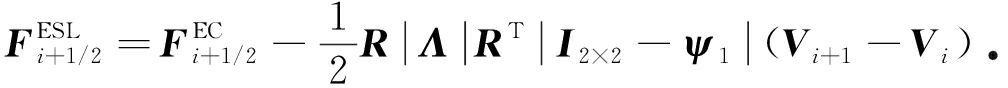(7)

## 2 二维浅水波方程求解

Ut+F(U)x+G(U)y=S

H(U)=guh2+(hu3+huv2)/2，K(U)=gvh2+(hv3+hvu2)/2,J=ghb[u,v]T

### 2.1熵守恒格式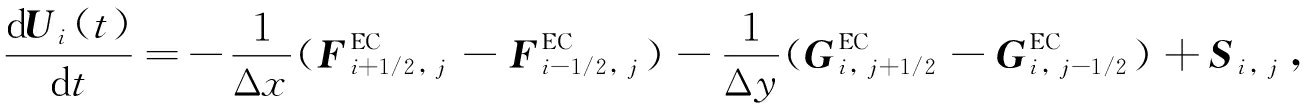(8)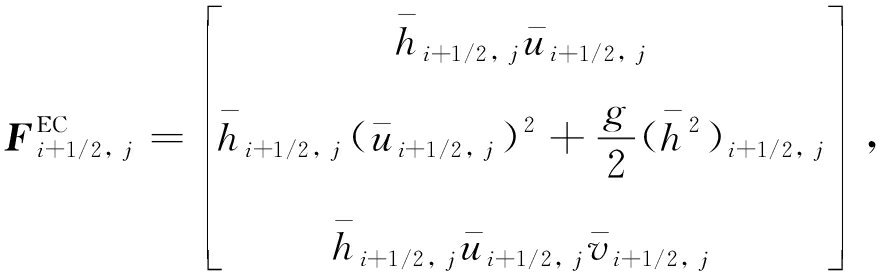(9)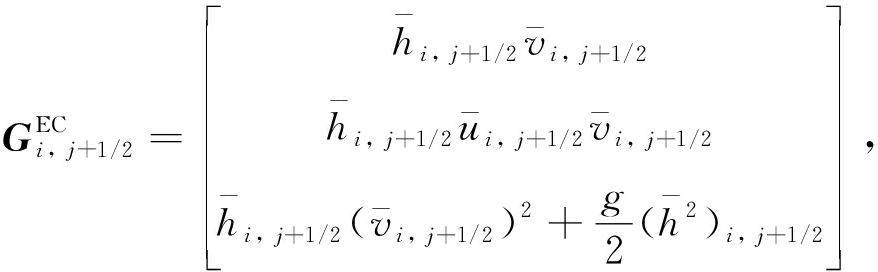(10)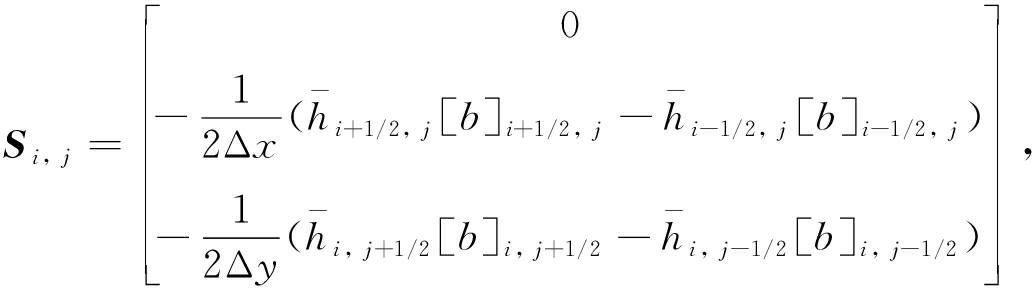(11)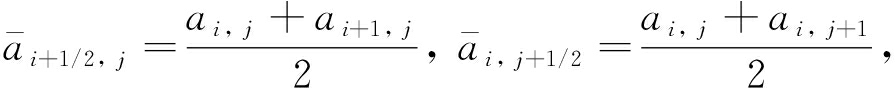[a]i+1/2,j=ai+1,j-ai,j， [a]i,j+1/2=ai,j+1-ai,j

### 2.2熵稳定格式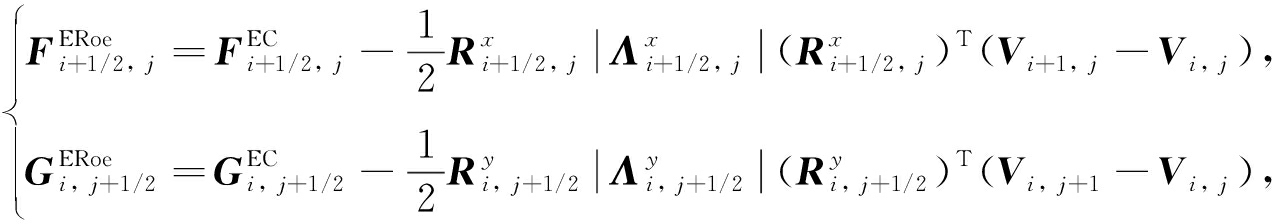(12)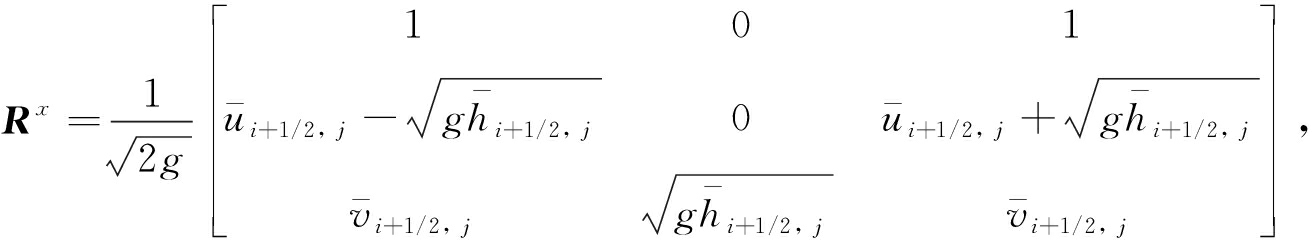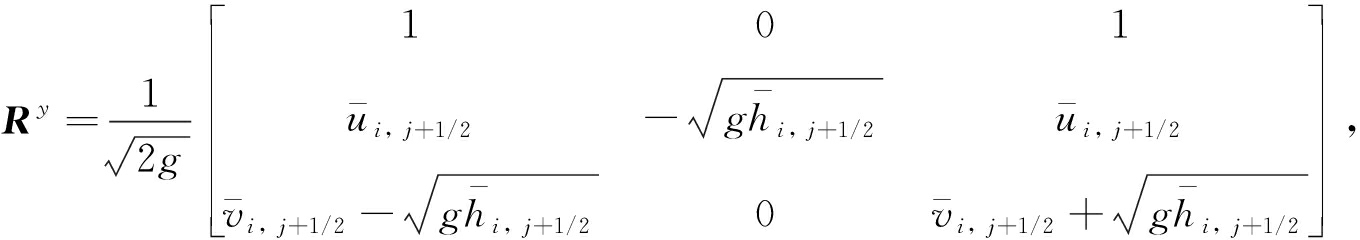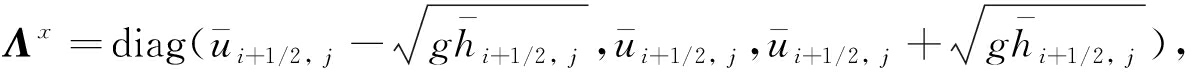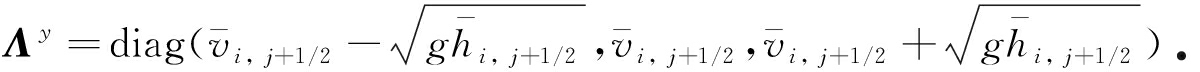### 2.3高分辨率的熵稳定格式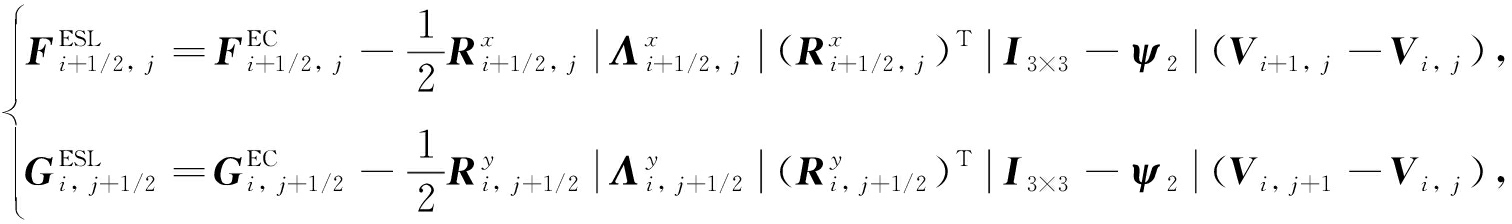(13)

## 3 数 值 算 例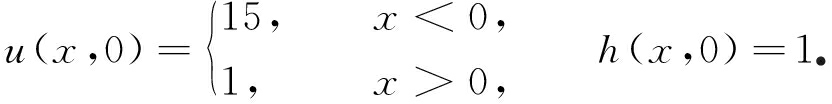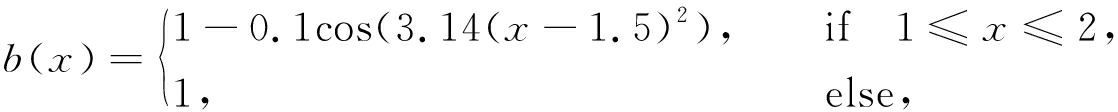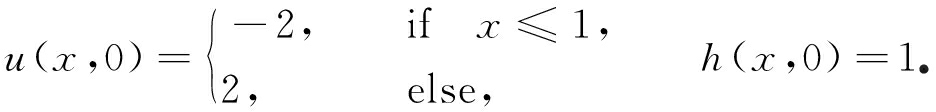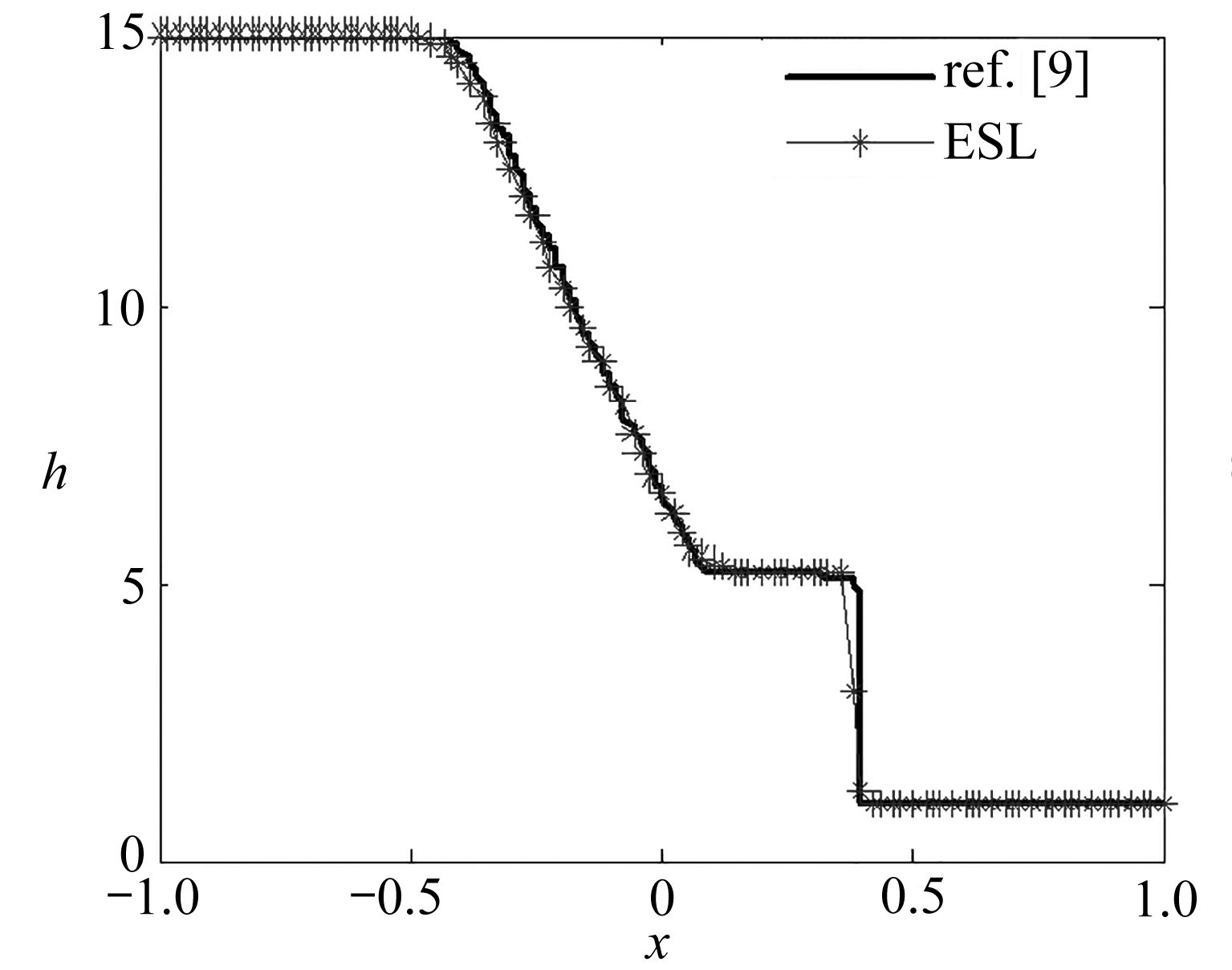(a) ESL格式
(a) Results with the ESL scheme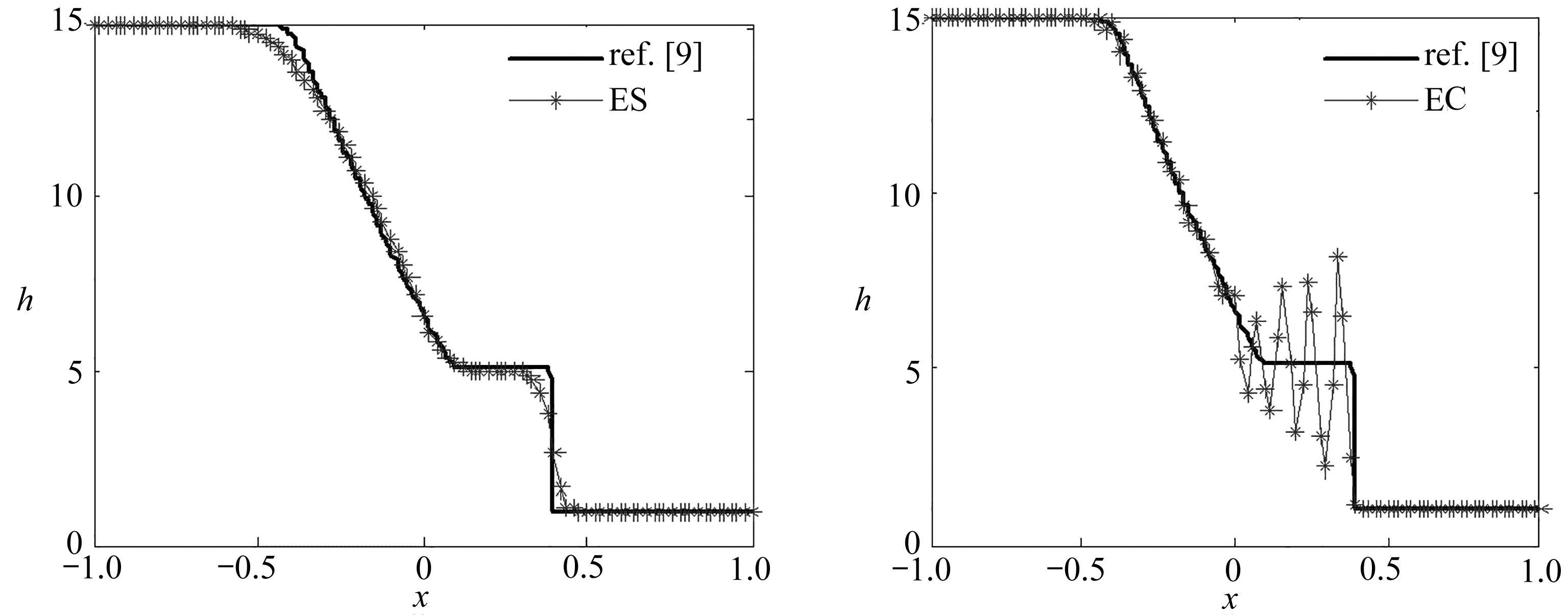(b) 熵稳定格式 (c) 熵守恒格式 (b) Results with the ES scheme (c) Results with the EC scheme

Fig. 1 Numerical results of a large dam break problem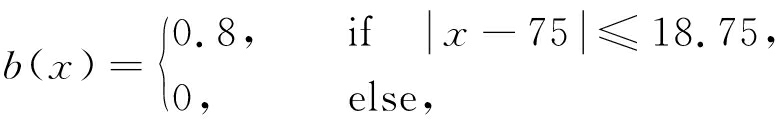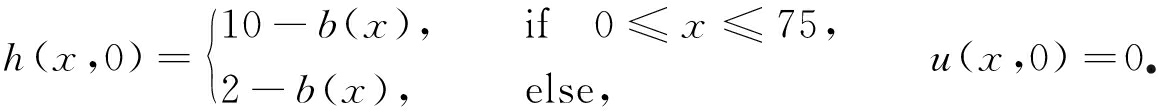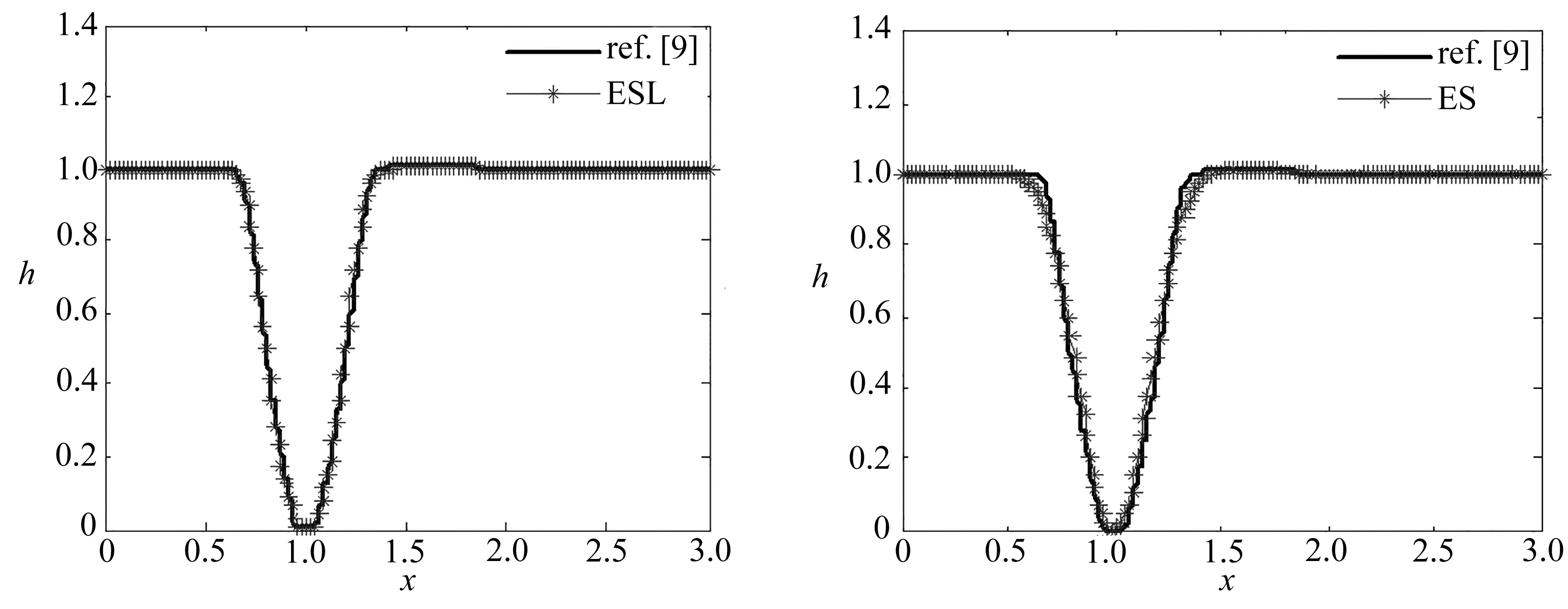(a) ESL格式 (b) 熵稳定格式 (a) Results with the ESL scheme (b) Results with the ES scheme

Fig. 2 Numerical results of a dam break problem of a near-zero height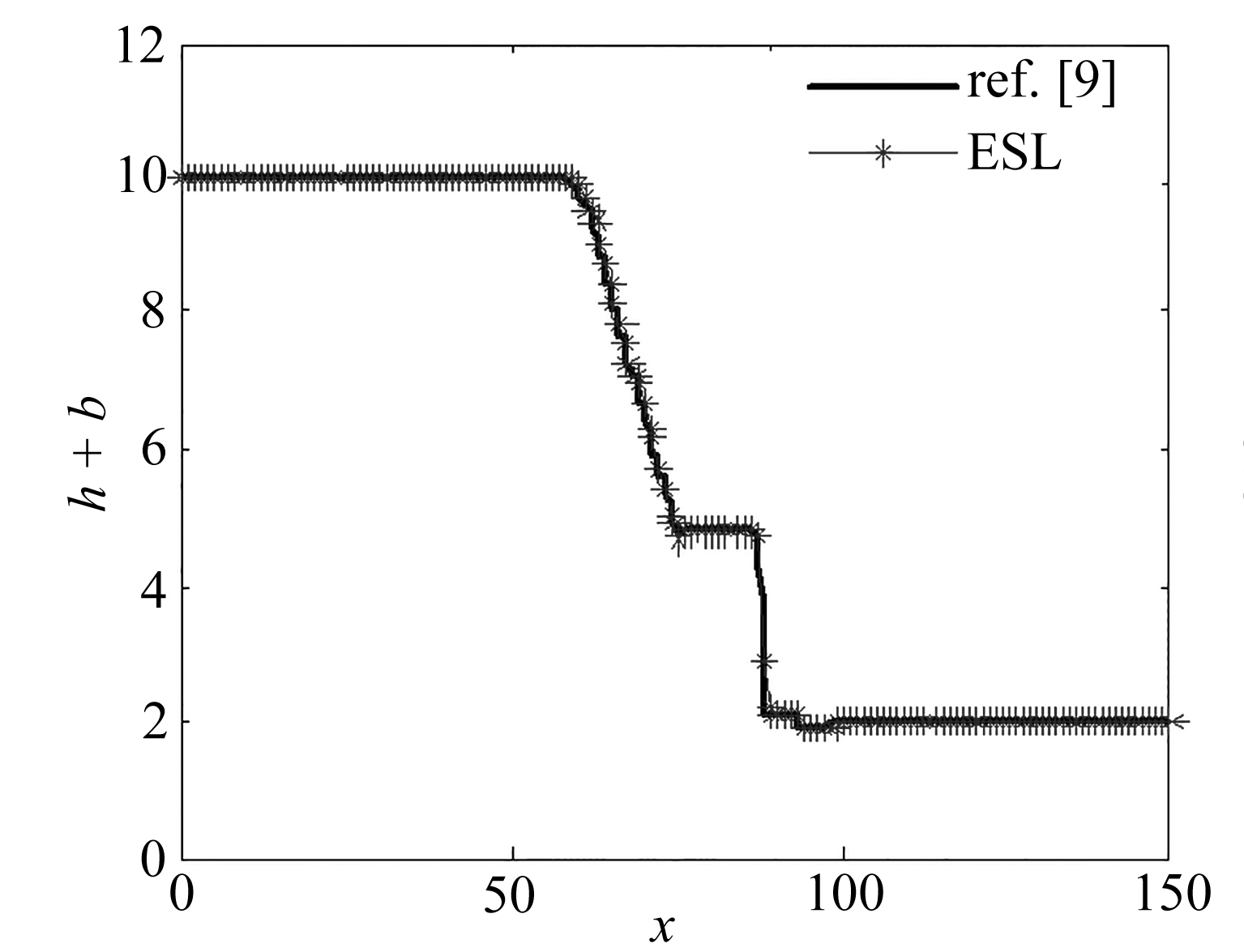(a) ESL格式
(a) Results with the ESL scheme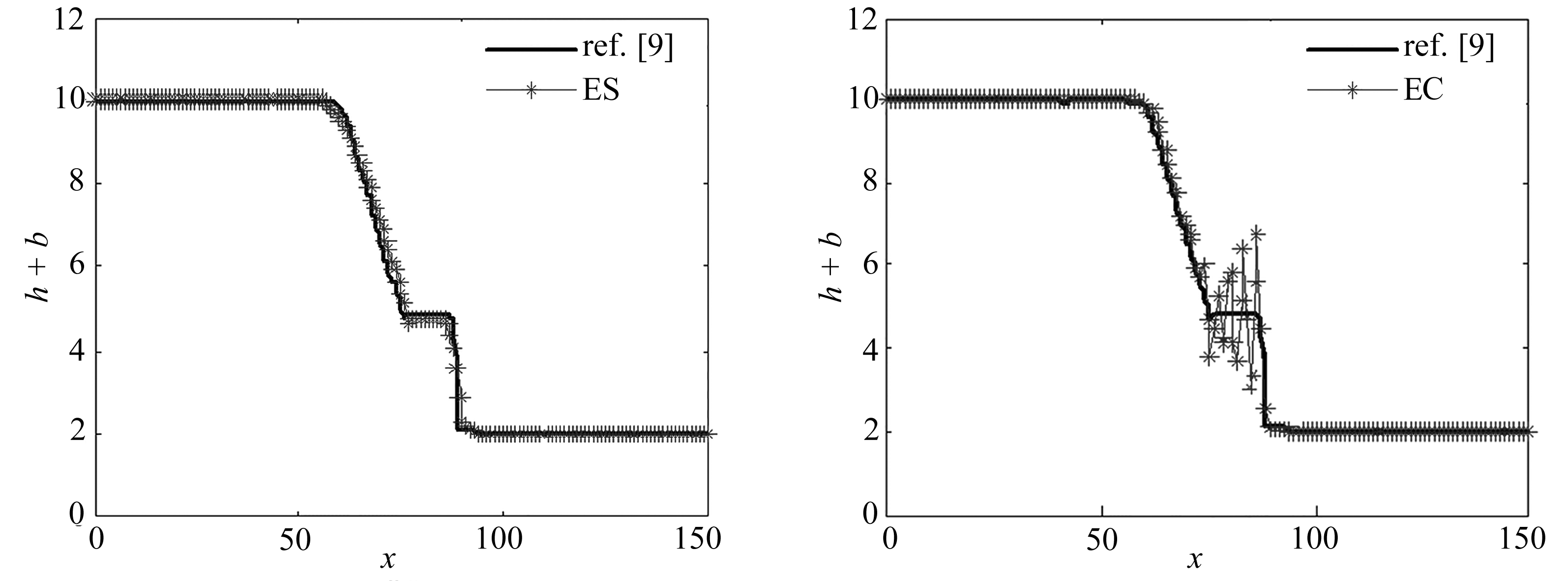(b) 熵稳定格式 (c) 熵守恒格式 (b) Results with the ES scheme (c) Results with the EC scheme

Fig. 3 Numerical results of the 1D dam break problem

b(x,y)=0.8e-50(x-0.9)2-50(y-0.5)2

u(x,y,0)=0，v(x,y,0)=0，h(x,y,0)=1-b(x,y)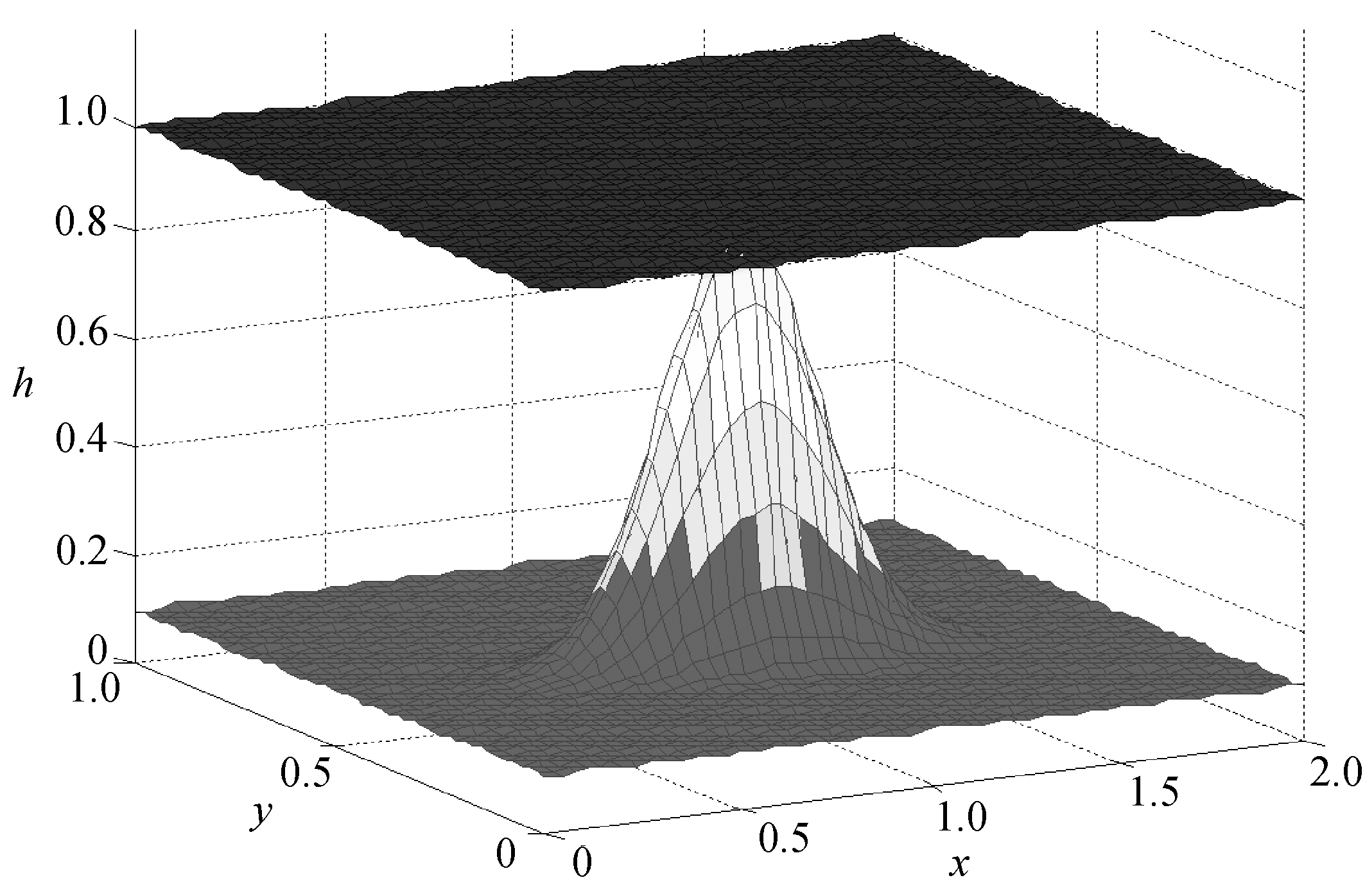Fig. 4 Results with the ESL scheme

Table 1L1errors and orders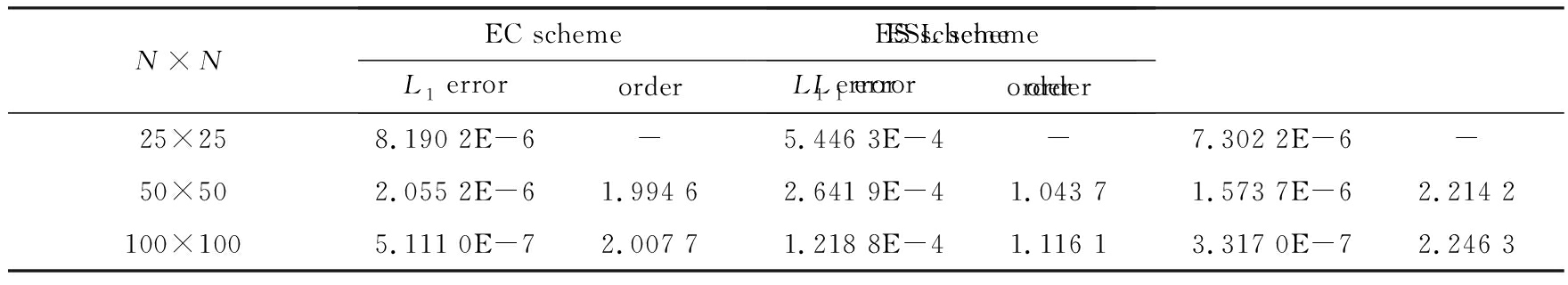Table 2L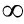errors and orders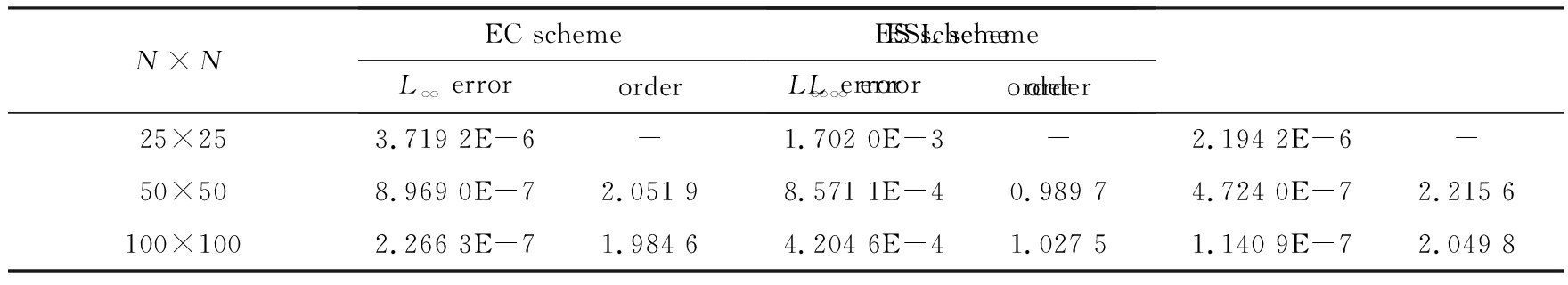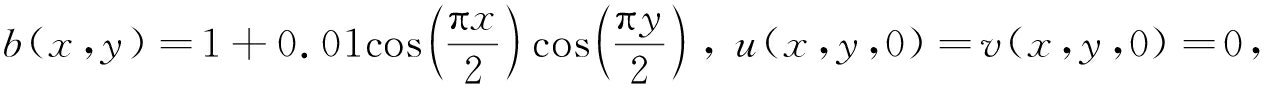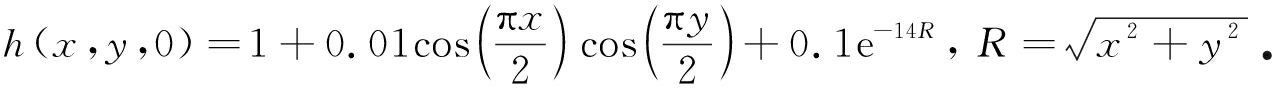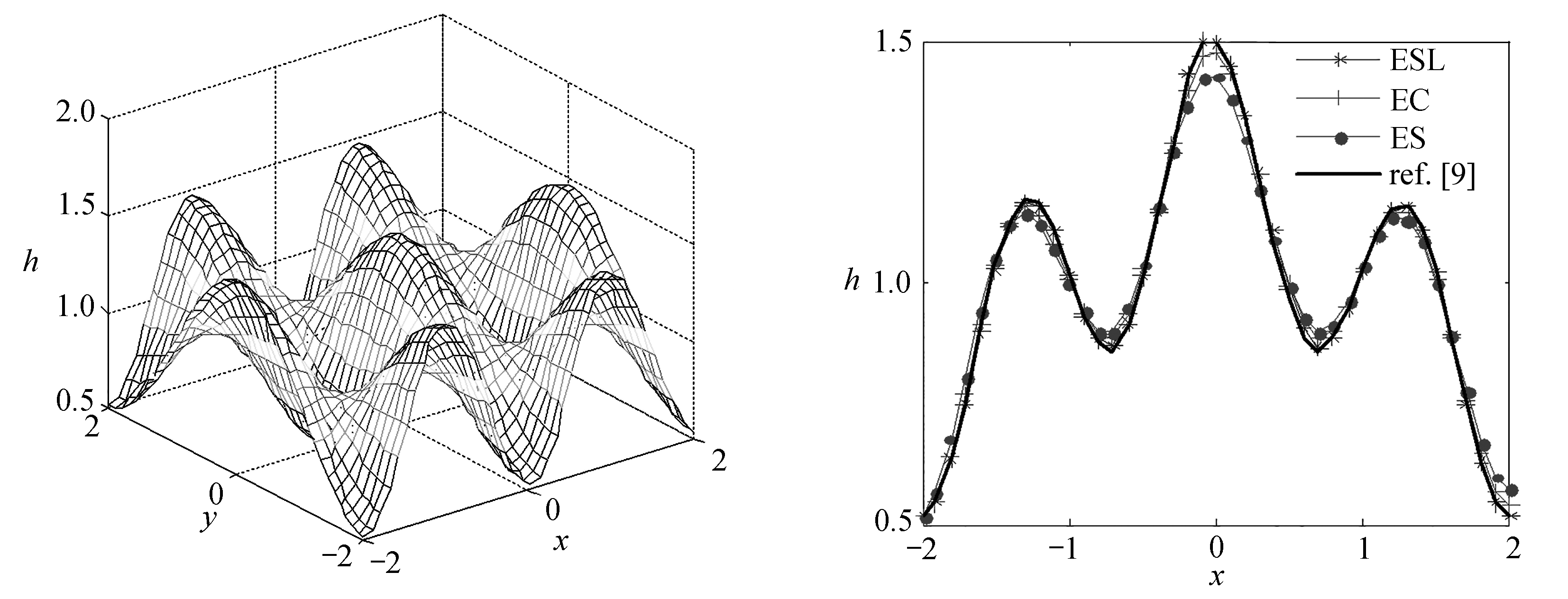(a) ESL格式的数值结果 (b) 截面y=x的水深数值结果 (a) Numerical results with ESL scheme (b) Numerical results of the water height at y=x

Fig. 5 Numerical results with the ESL scheme and the water height for the 2D wave equation

## 4 结 论

 FJORDHOLM U S, MISHRA S, TADMOR E. Well-balanced and energy stable schemes for the shallow water equations with discontinuous topography[J].Journal of Computational Physics, 2011,230(14): 5587-5609.

 LAX P D. Weak solutions of nonlinear hyperbolic equations and their numerical computation[J].Communications on Pure and Applied Mathematics, 1954,7(1): 159-193.

 LAX P D. Hyperbolic systems of conservation laws and the mathematical theory of shock waves[C]//SIAM Regional Conference Lectures in Applied Mathematics. Vol11. Philadelphia, USA, 1973.

 TADMOR E. The numerical viscosity of entropy stable schemes for systems of conservation laws. I[J].Mathematics of Computation, 1987,49(179): 91-103.

 ROE P L. Entropy conservation schemes forthe Euler equations[R]. Talk at HYP 2006, Lyon, France, 2006.

 ISMAIL F, ROE P L. Affordable, entropy-consistent Euler flux functions Ⅱ: entropy production at shocks[J].Journal of Computational Physics, 2009,228(15): 5410-5436.

 MOHAMMED A N, ISMAIL F. Study of an entropy-consistent Navier-Stokes flux[J].International Journal of Computational Fluid Dynamics, 2013,27(1): 1-14.

 LIU Y, FENG J, REN J. High resolution, entropy-consistent scheme using flux limiter for hyperbolic systems of conservation laws[J].Journal of Scientific Computing, 2015,64(3): 914-937.

 任炯, 封建湖, 刘友琼, 等. 求解双曲守恒律方程的高分辨率熵相容格式[J]. 计算物理, 2014,31(5): 539-551.(REN Jiong, FENG Jianhu, LIU Youqiong, et al. High resolution entropy consistent schemes for hyperbolic conservation laws[J].Chinese Journal of Computational Physics, 2014,31(5): 539-551.(in Chinese))

 刘友琼, 封建湖, 梁楠, 等. 求解浅水波方程的熵相容格式[J]. 应用数学和力学, 2013,34(12): 1247-1257.(LIU Youqiong, FENG Jianhu, LIANG Nan, et al. An entropy-consistent flux scheme for shallow water equations[J].Applied Mathematics and Mechanics, 2013,34(12): 1247-1257.(in Chinese))

 刘友琼, 封建湖, 任炯, 等. 求解多维Euler方程的二阶旋转混合型格式[J]. 应用数学和力学, 2014,35(5): 542-553.(LIU Youqiong, FENG Jianhu, REN Jiong, et al. A second-order rotated-hybrid scheme for solving multi-dimensional compressible Euler equations[J].Applied Mathematics and Mechanics, 2014,35(5): 542-553.(in Chinese))

 程晓晗, 聂玉峰, 蔡力. 基于WENO重构的熵稳定格式求解浅水方程[J]. 计算物理, 2015,32(5): 523-528.(CHENG Xiaohan, NIE Yufeng, CAI Li. WENO based entropy stable scheme for shallow water equations[J].Chinese Journal of Computational Physics, 2015,32(5): 523-528.(in Chinese))

 程晓晗, 封建湖, 聂玉峰. 求解双曲守恒律方程的WENO型熵相容格式[J]. 爆炸与冲击, 2014,34(4): 501-507.(CHENG Xiaohan, FENG Jianhu, NIE Yufeng. WENO type entropy consistent scheme for hyperbolic conservation laws[J].Explosion and Shock Waves, 2014,34(4): 501-507.(in Chinese))

 郑素佩, 封建湖, 刘彩侠. 高分辨率熵相容算法在二维溃坝问题中的应用[J]. 水动力学研究与进展, 2013,28(5): 545-551.(ZHENG Supei, FENG Jianhu, LIU Caixia. High-resolution entropy consistent algorithm for the two-dimensional dam-break flows[J].Chinese Journal of Hydrodynamics, 2013,28(5): 545-551.(in Chinese))

 GOTTLIEB S, SHU C W. A survey of strong stability preserving high order time discretizations[J].SIAM Review, 2001,43(1): 89-112.

 FJORHOLM U S. Structure preserving finite volume methods for the shallow water equations[D]. Master Thesis. Norway: University of Oslo, 2009.

# An Entropy Stable Scheme for Shallow Water Equations With Source Terms

ZHANG Haijun， FENG Jianhu， CHENG Xiaohan， LI Xue

(School of ScienceChangan UniversityXian710064，P.R.China)

Abstract:An entropy stable scheme was developed for the shallow water equations with source terms, and a 1st-order entropy stable scheme and a high-order entropy conservation scheme were combined with a flux limiter function. The new entropy scheme preserves advantages of both the entropy conservation scheme and the entropy stable scheme, having higher accuracy in the regions of the smooth solutions and capturing shocks accurately while avoiding non-physical phenomena in the regions of the discontinuous solutions, thus achieves high resolution. The new scheme was successfully applied to calculate the classical 1D and 2D problems. The numerical results show that the new scheme does be an ideal method to simulate the shallow water equations with source terms.

Key words:shallow water equations; entropy conservation scheme; entropy stable scheme; high resolution

Foundation item:The National Natural Science Foundation of China(11601037;11401045;11171043)

ⓒ 应用数学和力学编委会，ISSN 1000-0887

*收稿日期2017-07-13；

DOI:10.21656/1000-0887.380195